Home/Blog/Data structures 101: A deep dive into trees with Java

# Data structures 101: A deep dive into trees with Java

May 27, 2020 - 13 min read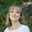Amanda FawcettData structures are an important part of programming and coding interviews. These skills show your ability to think complexly, solve ambiguous problems, and recognize coding patterns.

Programmers use data structures to organize data, so the more efficient your data structures are, the better your programs will be.

Today, we will take a deep dive into one of the most popular data structures out there: trees.

Today, we will cover:

## What is a tree?

Data structures are used to store and organize data. We can use algorithms to manipulate and use our data structures. Different types of data are organized more efficiently by using different data structures.

Trees are non-linear data structures. They are often used to represent hierarchical data. For a real-world example, a hierarchical company structure uses a tree to organize.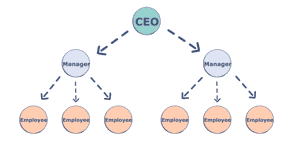### Components of a Tree

Trees are a collection of nodes (vertices), and they are linked with edges (pointers), representing the hierarchical connections between the nodes. A node contains data of any type, but all the nodes must be of the same data type. Trees are similar to graphs, but a cycle cannot exist in a tree. What are the different components of a tree?

Root: The root of a tree is a node that has no incoming link (i.e. no parent node). Think of this as a starting point of your tree.

Children: The child of a tree is a node with one incoming link from a node above it (i.e. a parent node). If two children nodes share the same parent, they are called siblings.

Parent: The parent node has an outgoing link connecting it to one or more child nodes.

Leaf: A leaf has a parent node but has no outgoing link to a child node. Think of this as an endpoint of your tree.

Subtree: A subtree is a smaller tree held within a larger tree. The root of that tree can be any node from the bigger tree.

Depth: The depth of a node is the number of edges between that node and the root. Think of this as how many steps there are between your node and the tree’s starting point.

Height: The height of a node is the number of edges in the longest path from a node to a leaf node. Think of this as how many steps there are between your node and the tree’s endpoint. The height of a tree is the height of its root node.

Degree: The degree of a node refers to the number of sub-trees.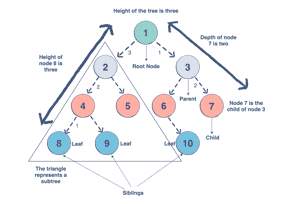### Why do we use trees?

Trees can be applied to many things. The hierarchical structure gives a tree unique properties for storing, manipulating, and accessing data. Trees form some of the most basic organization of computers. We can use a tree for the following:

• Storage as hierarchy. Storing information that naturally occurs in a hierarchy. File systems on a computer and PDF use tree structures.
• Searching. Storing information that we want to search quickly. Trees are easier to search than a Linked List. Some types of trees (like AVL and Red-Black trees) are designed for fast searching.
• Inheritance. Trees are used for inheritance, XML parser, machine learning, and DNS, amongst many other things.
• Indexing. Advanced types of trees, like B-Trees and B+ Trees, can be used for indexing a database.
• Networking. Trees are ideal for things like social networking and computer chess games.
• Shortest path. A Spanning Tree can be used to find the shortest paths in routers for networking.
• and much more

### How to make a tree

But, how does that all look in code? To build a tree in Java, for example, we start with the root node.

Node<String> root = new Node<>("root");


Once we have our root, we can add our first child node using addChild, which adds a child node and assigns it to a parent node. We refer to this process as insertion (adding nodes) and deletion (removing nodes).

Node<String> node1 = root.addChild(new Node<String>("node 1"));


We continue adding nodes using that same process until we have a complex hierarchical structure. In the next section, let’s look at the different kinds of trees we can use.

## Types of Trees

There are many types of trees that we can use to organize data differently within a hierarchical structure. The tree we use depends on the problem we are trying to solve. Let’s take a look at the trees we can use in Java. We will be covering:

• N-ary trees
• Balanced trees
• Binary trees
• Binary Search Trees
• AVL Trees
• Red-Black Trees
• 2-3 Trees
• 2-3-4 Trees

### N-ary Tree

In N-ary tree, a node can have child nodes from 0-N. For example, if we have a 2-ary tree (also called a Binary Tree), it will have a maximum of 0-2 child nodes.

N-ary tree

Note: The balance factor of a node is the height difference between the left and right subtrees.

### Balanced Tree

A balanced tree is a tree with almost all leaf nodes at the same level, and it is most commonly applied to sub-trees, meaning that all sub-trees must be balanced. In other words, we must make the tree height balanced, where the difference between the height of the right and left subtrees do not exceed one. Here is a visual representation of a balanced tree.

Binary tree

There are three main types of binary trees based on their structures.

#### 1. Complete Binary Tree

A complete binary tree exists when every level, excluding the last, is filled and all nodes at the last level are as far left as they can be. Here is a visual representation of a complete binary tree.

Complete Binary Tree (left side is completely filled)

#### 2. Full Binary Tree

A full binary tree (sometimes called proper binary tree) exits when every node, excluding the leaves, has two children. Every level must be filled, and the nodes are as far left as possible. Look at this diagram to understand how a full binary tree looks.

a full binary tree

#### 3. Perfect Binary Tree

A perfect binary tree should be both full and complete. All interior nodes should have two children, and all leaves must have the same depth. Look at this diagram to understand how a perfect binary tree looks.

a perfect binary tree

Note: You can also have a skewed binary tree, where all the nodes are shifted to the left or right, but it is best practice to avoid this type of tree in Java, as it is far more complex to search for a node.

### Binary Search Trees

A Binary Search Tree is a binary tree in which every node has a key and an associated value. This allows for quick lookup and edits (additions or removals), hence the name “search”. A Binary Search Tree has strict conditions based on its node value. It’s important to note that every Binary Search Tree is a binary tree, but not every binary tree is a Binary Search Tree.

What makes them different? In a Binary Search Tree, the left subtree of a subtree must contain nodes with fewer keys than a node’s key, while the right subtree will contain nodes with keys greater than that node’s key. Take a look at this visual to understand this condition.

In this example, the node Y is a parent node with two child nodes. All nodes in subtree 1 must have a value less than node Y, and subtree 2 must have a greater value than node Y.

### AVL Trees

AVL trees are a special type of Binary Search tree that are self-balanced by checking the balance factor of every node. The balance factor should either be +1, 0, or -1. The maximum height difference between the left and right sub-trees can only be one.

If this difference becomes more than one, we must re-balance our tree to make it valid using rotation techniques. These are most common for applications where searching is the most important operation. Look at this visual to see a valid AVL tree.

### Red-Black Trees

A red-black tree is another type of self-balancing Binary Search Tree, but it has some additional properties to AVL trees. The nodes are colored either red or black to help re-balance a tree after insertion or deletion. They save you time with balancing. So, how do we color our nodes?

• The root is always black
• Two red nodes cannot be adjacent (i.e. a red parent cannot have a red child)
• A path from the root to a leaf should contain the same number of black nodes
• A null node is black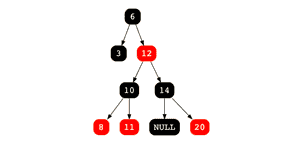a valid red-black tree

### 2-3 Trees

A 2-3 tree is very different from what we’ve learned so far. Unlike a Binary Search Tree, a 2-3 Tree is a self-balancing, ordered, multiway search tree. It is always perfectly balanced, so every leaf node is equidistant from the root. Every node, other than leaf nodes, can be either a 2-Node (a node with a single data element and two children) or a 3-node (a node with two data elements and three children). A 2-3 tree will remain balanced no matter how many insertions or deletions occur.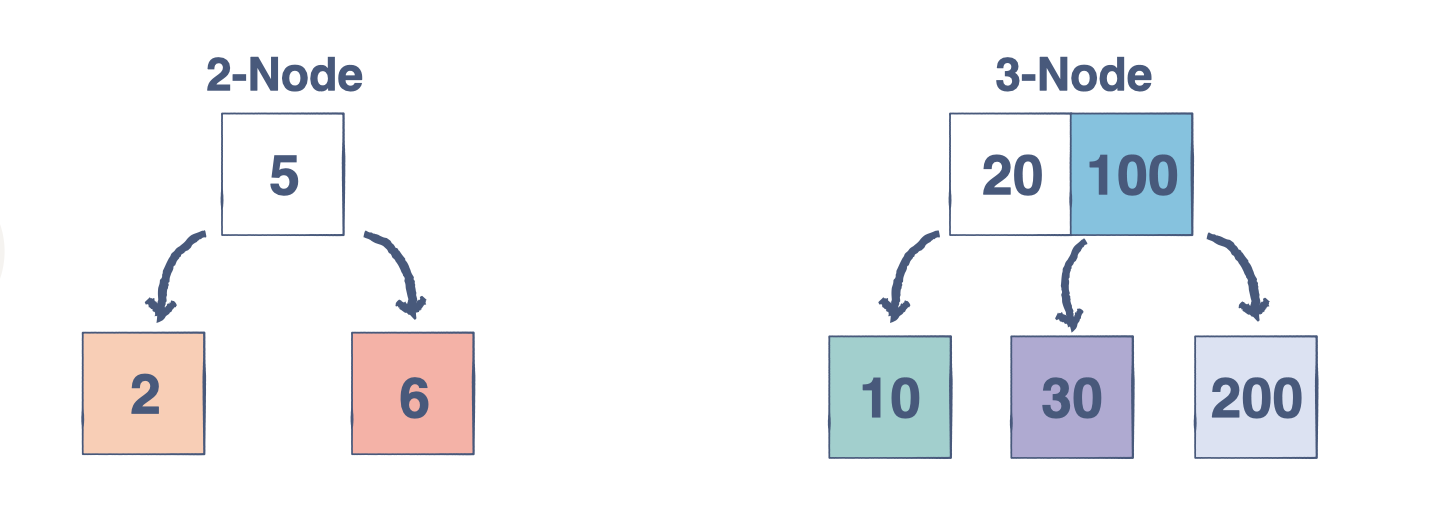### 2-3-4 Trees

A 2-3-4 tree is a search tree that can accommodate more keys than a 2-3 tree. It covers the same basics as a 2-3 tree, but adds the following properties:

• 2-Node has two child nodes and one data element
• 3-Node has three child nodes and two data elements
• 4-Node has four child nodes and three data elements
• Each internal node has a max of 4 children
• For three keys at an internal node, all keys at the LeftChild node are smaller than the left key
• All keys at the LeftMidChild are smaller than the mid key
• All keys at the RightMidChild are smaller than the right key
• All keys at the RightChild are greater than the right key#### Keep the learning going.

Learn data structures and algorithms in Java without scrubbing through videos or documentation. Educative’s text-based courses are easy to skim and feature live coding environments - making learning quick and efficient.

Algorithms for Coding Interviews in Java

## Intro to Tree Traversal and Searching

To use trees, we can traverse them by visiting/checking every node of a tree. If a tree is “traversed”, this means every node has been visited. There are four ways to traverse a tree. These four processes fall into one of two categories: breadth-first traversal or depth-first traversal.

• Inorder: Think of this as moving up the tree, then back down. You traverse the left child and its sub-tree until you reach the root. Then, traverse down the right child and its subtree. This is a depth-first traversal.

• Preorder: This of this as starting at the top, moving down, and then over. You start at the root, traverse the left sub-tree, and then move over to the right sub-tree. This is a depth-first traversal.

• Postorder: Begin with the left-sub tree and move over to the right sub-tree. Then, move up to visit the root node. This is a depth-first traversal.

• Levelorder: Think of this as a sort of zig-zag pattern. This will traverse the nodes by their levels instead of subtrees. First, we visit the root and visit all children of that root, left to right. We then move down to the next level until we reach a node that has no children. This is the left node. This is a breadth-first traversal.

So, what’s the difference between a breadth-first and depth-first traversal? Let’s take a look at the algorithms Depth-First Search (DFS) and Breath-First Search (BFS) to understand this better.

Note: Algorithms are a sequence of instructions for performing certain tasks. We use algorithms with data structures to manipulate our data, in this case, to traverse our data.

### Depth-First Search

Overview: We follow a path from the starting node to the ending node and then start another path until all nodes are visited. This is commonly implemented using stacks, and it requires less memory than BFS. It is best for topographical sorting, such as graph backtracking or cycle detection.

The steps for the DFS algorithm are as follows:

1. Pick a node. Push all adjacent nodes into a stack.
2. Pop a node from that stack and push adjacent nodes into another stack.
3. Repeat until the stack is empty or you have reached your goal. As you visit nodes, you must mark them as visited before proceeding, or you will be stuck in an infinite loop.

Overview: We proceed level-by-level to visit all nodes at one level before going to the next. The BFS algorithm is commonly implemented using queues, and it requires more memory than the DFS algorithm. It is best for finding the shortest path between two nodes.

The steps for the BFS algorithm are as follows:

1. Pick a node. Enqueue all adjacent nodes into a queue. Dequeue a node, and mark it as visited. Enqueue all adjacent nodes into another queue.
2. Repeat until the queue is empty of you have met your goal.
3. As you visit nodes, you must mark them as visited before proceeding, or you will be stuck in an infinite loop.

### Search in Binary Search Trees

It’s important to know how to perfom a search in a tree. Searching means we are locating a specific element or node in our data structure. Since data in a Binary Search Tree is ordered, searching is quite easy. Let’s see how it’s done.

1. Start at the root.
2. If the value is less than the value of the current node, we traverse the left sub-tree. If it is more, we traverse the right sub-tree.
3. Continue this process until you reach a node with that value or reach a leaf node, meaning that the value doesn’t exist.

In the below example, we are searching for the value 3 in our tree. Take a look.

Here is our tree. Since 3 is less than 5, we traverse the left sub-tree.
Since 3 is more than 2, we move to the right child.
The searched value has been found.

Let’s see that in Java code now!

public class BinarySearchTree {
…

public boolean search(int value) {
if (root == null)
return false;
else
return root.search(value);
}
}
public class BSTNode {
…

public boolean search(int value) {
if (value == this.value)
return true;
else if (value < this.value) {
if (left == null)
return false;
else
return left.search(value);
} else if (value > this.value) {
if (right == null)
return false;
else
return right.search(value);
}
return false;
}
}

## Wrapping up and Next Steps

Congratulations on completing your next step into the world of Java and data structures. However, there are still many interview questions and data structures to practice.

Here are some of the common data structures challenges you should look into to get a better sense of how to use trees:

• Find the minimum value of a Binary Search Tree
• Find the ancestors of a given node in a Binary Search Tree
• Find the height of a Binary Tree
• Find nodes at “k” distance from the root
• and more

To get started learning these challenges, check out Data Structures for Coding Interviews in Java, which breaks down all the data structures common to Java interviews alongside hands-on, interactive quizzes and coding challenges.

This course gets you up-to-speed on all the fundamentals of computer science in an accessible, personalized way.